International Journal of Energy and Power Engineering
Volume 5, Issue 1-1, February 2016, Pages: 31-36

Computation and Analysis of the DC-Biasing Magnetic Field by the Harmonic-Balanced Finite-Element Method

Xiaojun Zhao1, Dawei Guan1, Fanhui Meng1, Yuting Zhong1, and Zhiguang Cheng2

1Department of Electrical Engineering, North China Electric Power University, Baoding, China

2Institute of Power Transmission and Transformation Technology, Baobian Electric Co., Ltd, Baoding, China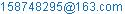(Xiaojun Zhao)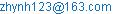(Dawei Guan)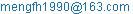(Fanhui Meng)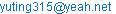(Yuting Zhong)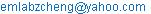(Zhiguang Cheng)

Xiaojun Zhao, Dawei Guan, Fanhui Meng, Yuting Zhong, Zhiguang Cheng. Computation and Analysis of the DC-Biasing Magnetic Field by the Harmonic-Balanced Finite-Element Method. International Journal of Energy and Power Engineering. Special Issue: Numerical Analysis, Material Modeling and Validation for Magnetic Losses in Electromagnetic Devices. Vol. 5, No. 1-1, 2016, pp. 31-36. doi: 10.11648/j.ijepe.s.2016050101.14

Abstract: This paper sought to present harmonic-balanced method for finite element analysis of nonlinear eddy current field. The harmonic-balanced method can be used to compute the time-periodic electromagnetic field in harmonic domain, considering electric circuits coupled with the nonlinear magnetic field. 2-D and simplified 3-D model of laminated core is established and computed to prove the accuracy and validity of the proposed method. The calculated magnetizing current is compared with the measured results and the computed magnetic field is analyzed to investigate the effect of DC bias.

Keywords: Eddy Current, Finite Element Analysis, Harmonic Domain, Time-Periodic

1. Introduction

The DC bias phenomenon is an abnormal operation state of the power transformer. The direct current flows into the windings of transformers, which may lead to a series of problems in transformers and electric networks. Such problems include significant saturation of the ferromagnetic core, vibration and overheating of transformers -, and reactive power demand  in the transmission system. The investigation of the DC biased problem originates from the effect of Geomagnetically Induced Current (GIC) - caused by solar magnetic disturbance on transformers and electric networks. With the development of high voltage direct current (HVDC) transmission, more attention has been paid to the DC biased problem in recent years. In the monopolar operating mode of the HVDC system, the earth is usually used as a return path -. In that case, there will be a large DC potential difference between the two converting plants. The electric potential difference generates a direct current that flows into the windings of the power transformer (through the earthed neutrals in the AC network).

Research on the mechanism of the DC biased problem contributes to important developments in transformer design. Many different methods have been used to investigate the electromagnetic field in transformers under the DC bias condition -. The circuit model - were proposed to calculate the excitation current in windings. The time-stepping finite element method  was also used by some researchers to compute the magnetizing current and magnetic field. However, it is difficult to obtain accurate results from the electric or magnetic circuit model, especially when magnetic field analysis is required to explore the mechanism of the DC biased problem. The time-stepping method is an alternative method to calculate the transient magnetic field, but accurate solutions of high order harmonic components in exciting current and magnetic induction require many more iterations in the time domain, which can reduce the effectiveness of this method.

In this paper the HBFEM is used to solve the nonlinear magnetic field under different DC bias conditions, considering the coupling of the electric circuit and the magnetic field. Harmonic solutions of the magnetizing current and the magnetic field can be calculated directly and quickly in the harmonic domain. The nonlinear magnetic field under DC bias condition is analyzed thoroughly through harmonic analysis in order to investigate the mechanism of the DC bias phenomenon in the power transformer. The calculated results and experimental data are compared on an Epstein frame-like core model, which proves that the HBFEM is an effective and efficient method in analyzing the DC biased problem of power transformers.

2. Harmonic-Balanced Finite-Element Method

2.1. Harmonic Balance Method in Finite Element Analysis of Nonlinear Magnetic Field

The nonlinear magnetic field can be described by using magnetic vector potential A,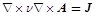(1)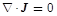(2)

where ν is the reluctivity, J is the current density including exciting current density J0 and eddy current density Je.

The finite element equation can be obtained by using Galerkin’s method,(3)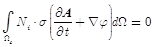(4)

where Ni is the nodal shape function, φ is the electric scalar potential, Ωn and Ωc are the finite element in the whole domain and non-conducting domain, respectively. σ is the conductivity of conducting materials. The penalty function is always required in finite element analysis of eddy current problems based on nodal basis function .

The time-periodic solutions are focused in the DC bias phenomenon, since it is a harmonic problem with alternating and direct excitations. The magnetic flux density and magnetic vector potentials are both periodic functions in the time domain. Based on harmonic balance theory, all variables in (3) can be expressed by complex series as follows,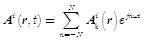(5)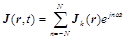(6)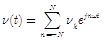(7)

where N is the truncated harmonic number.

Considering the orthogonal characteristics of trigonometric functions, the harmonic-balanced equation can be attained as follows,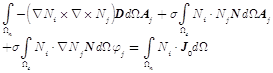(8)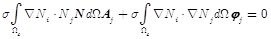(9)

where Aj, φj are harmonic solutions, D and N are named as reluctivity matrix and harmonic matrix respectively,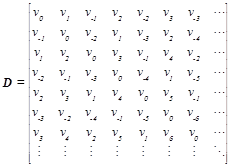(10)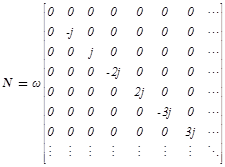(11)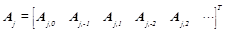(12)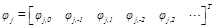(13)

2.2. Harmonic-Balanced Method in Electric Circuits Coupled to Magnetic Field

Most of electromagnetic devices are connected to the voltage source and therefore electric circuits coupled with magnetic field should be considered.

According to Kirchhoff’s Law the applied voltage on the external port of the electric circuit can be defined as follows,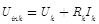(14)

where Uink is the input voltage of circuit k, and Uk is the corresponding induced electromotive force.

The induced electromotive force can be obtained in (15) based on Faraday’s Law,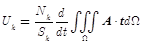(15)

where t is the current vector representing the flowing direction of the exciting current.

Consequently we can attain the harmonic-balanced equation of the electromagnetic coupling as follows,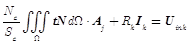(16)

where k indicate the circuit number, Nc is the number of turns and Sc is the cross-section area of the exciting coil. Aj and Ik are the harmonic solutions of the magnetic vector potential and exciting current, respectively.

Finally the harmonic-balanced system equation can be represented simply as follows,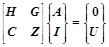(17)

3. Computational Results and Field Analysis

3.1. Laminated Core Under DC-Biased Condition

The Epstein frame-like core model for the DC biased test is shown in Fig. 1. The iron core is made up of silicon steel lamination of which the model number is 30Q140. There are two windings on the ferromagnetic core: the exciting coil (connected to alternating voltage source) and the measuring coil.

The peak value of excitation current without a DC bias is selected as a reference. This reference current causes the flux density in the silicon steel to reach the rated value (1.7T) in the transformer’s no-load operation. The DC bias in the form of direct current is then applied in proportion to the reference current to the exciting coil.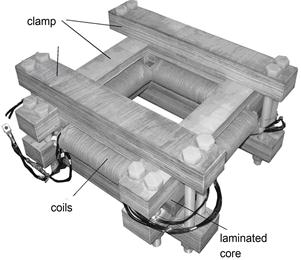Figure 1. Laminated Core Model Used in DC-Biased Experiment.

The value of reference current I0 measured on the square ferromagnetic core model is 1.68 ampere. The DC bias is applied in incremental proportions of the reference current, which are represented by Pi (i=1, 2, 3, 4) in Table 1. The AC excitation is also applied in four different cases indicated by the subscript j (j=1, 2, 3, 4). Idc represents the DC bias current that corresponds to different proportions of the reference current I0, while Hdc is the subsequently generated magnetic intensity. The peak value of alternating flux density Bm in the magnetic core varies with the step-increase of alternating voltage Um (peak value) , which is also shown in the same Table.

Table 1. Different DC bias condition by quantity in the magnetic field.

 Cases (i/j) DC bias AC excitation Pi ( %I0) Idc,i(A) Hdc,i(A/m) Um,j(V) Bm,j( T) 1 25 0.4256 105.68 26 0.09 2 50 0.847 213.12 133 0.49 3 75 1.273 320.30 240 0.88 4 100 1.697 425.23 370 1.37 5 150 2.530 636.58 420 1.57 6 495 1.82

3.2. Computational Results and Analysis

3.2.1. Calculated Exciting Currents and Measured Results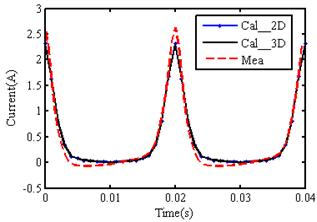Figure 2. Comparison of magnetizing currents between calculation and measurement (Um = Um,3 = 240V, Bm = Bm,3 = 0.88T).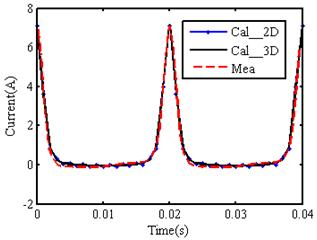Figure 3. Comparison of magnetizing currents between calculation and measurement (Um = Um,4= 370V, Bm = Bm,4 = 1.37T).

Harmonic solutions of the exciting currents and magnetic vector potential are computed simultaneously in (17). The calculated currents are compared with the measured data. As shown in Fig. 2 and Fig. 3, there is consistency in the computational and measured results obtained from the magnetizing current waveforms.

3.2.2. Harmonic Analysis of the Magnetizing Current

There are only odd harmonics in the magnetizing current when the transformer is fed by AC excitation. However, additional harmonics appear when the direct current invades the transformer windings. The generation of large harmonics results in significant saturation of the magnetic core and half-cycle saturation of the magnetizing current. Therefore, the relationship between the DC bias and harmonic components should be considered by using harmonic analysis .

Unlike the time-domain iterations and the Fourier transforming process of the solution in the time-stepping finite element method, all harmonic components in the magnetizing current can be obtained directly from the harmonic solution using the HBFEM. The histograms in Fig.4 and Fig.6 show the contribution of different harmonic components to the magnetizing current under different DC biases.

Fig. 4 shows that while the size of all harmonic components increases when additional DC bias is applied, the growth rate varies in different components. The growth tendency of each harmonic is shown in Fig. 5. The numbers 1, 2, 3, 4 in the horizontal coordinate represent different proportions (25%, 50%, 75%, 100%) of the DC bias reference current respectively. It is obvious that the fundamental and second harmonic components increase near-linearly, while higher order harmonics (the third and fourth) grow faster rather than linearly.

The contribution of each harmonic component is different when the peak value of alternating voltage is increased up to 495 volts, which is given in Fig. 6. Odd harmonics are greater than even order components under 25% and 50% DC bias respectively. It is implied that the growth of odd harmonic components is related to the increased AC excitation.

Curves in Fig. 7 display a relationship between odd harmonics and AC excitation. With the increased alternating voltage odd harmonics grow faster (and are greater in size) than the even harmonics. On the other hand, the negative influence of DC bias on each harmonic is analysed in Fig. 8 when the ferromagnetic core is significantly saturated as a consequence of high alternating voltage. Even harmonics increase faster than the odd harmonics with the increased DC bias and constant AC excitation.

It can be concluded that the appearance of DC bias in exciting current leads to the generation of even harmonics in the DC biased problem, and each harmonic component in the exciting current is affected by DC and AC excitation simultaneously. The applied alternating voltage makes the main contribution to the growth of odd harmonics while the DC bias plays a more important role in the variation of even harmonics, especially when the ferromagnetic core is significantly saturated.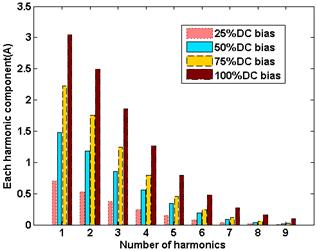Figure 4. Each harmonic component of exciting current under different DC bias (Um = Um,3 = 240V; Bm = Bm,3 = 0.88T).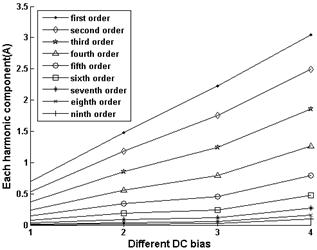Figure 5. DC bias effect on different harmonics (Um = Um,3 = 240V; Bm = Bm,3 = 0.88T).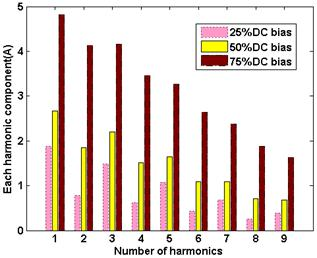Figure 6. Each harmonic component of exciting current under different DC bias (Um = Um,6 = 495V; Bm = Bm,6 = 1.82T).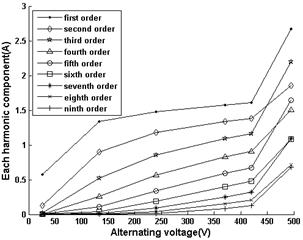Figure 7. AC voltage (peak value) effect on each harmonic component under 50% DC bias (Idc = Idc,2= 0.847A; Hdc = Hdc,2 = 213.12A/m).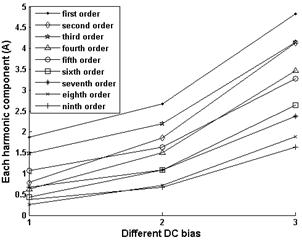Figure 8. DC bias effect on different harmonics (Um = Um,6 = 495V; Bm = Bm,6 = 1.82T).

3.2.3. Calculated Magnetic Field

The averaged flux density in the laminated core is attained from the harmonic solutions of vector potential. As shown in Fig. 9, flux density in the laminated core calculated in 2-D model agrees well with that in 3-D model.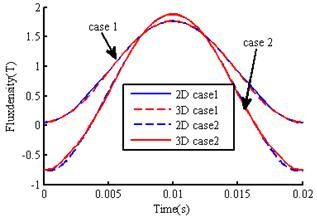Figure 9. Flux density in the laminated core computed in 2-D and 3-D model (case 1:Um = Um,3 = 240V, Bm = Bm,3 = 0.88T; case 2:Um = Um,4 = 370V, Bm = Bm,4 = 1.37T;).

Harmonic flux distributions under DC bias condition are presented in Fig.10. The harmonic flux distributions vary with time (related to phase angle) and excitations (related to DC bias and alternating voltage) .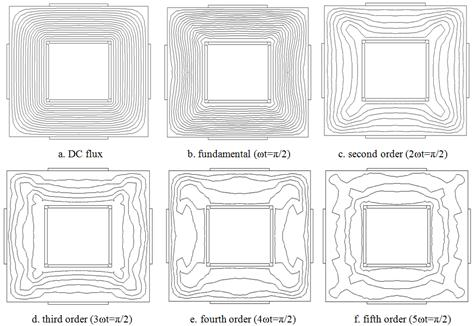Figure 10. Harmonic flux distributions (Idc=1.27A, Um= Um,4 =370V).

4. Conclusion

The time-periodic magnetic field can be computed in harmonic domain by the harmonic-balanced finite-element method. The time-stepping method requires many periods to approach the steady state solution in time domain, instead, the harmonic-balanced method computes nonlinear magnetic field directly in frequency domain. Harmonic solutions can be decoupled by the decomposed algorithm  and the system equation is suitable for concurrent computation. It can be concluded that the harmonic-balanced method requires less memory for the solution since the number of harmonics is usually less than the number of time steps in computation.

Acknowledgement

This work is supported by the National Natural ScienceFoundation of China (Grant No. 51307057), Hebei Province Natural Science Foundation (Grant No.E2013502323), Research Fund for the Doctoral Program of Higher Education of China (Grant No. 20130036120011), and the Fundamental Research Funds for the Central Universities (Grant No. 2015MS82).

References

1. P. Picher, L. Bolduc, A. Dutil, and V. Q. Pham, "Study of the acceptable DC current limit in core-form power transformers," IEEE Trans. Power Del., vol. 12, no. 1, pp. 257–265, Jan. 1997.
2. H. C. Tay and G. W. Swift, "On the problem of transformer overheating due to geomagnetically induced current," IEEE Trans. Power App. Syst., vol. PAS-104, no. 1, pp. 212–219, Jan. 1985.
3. Y. You, E. F. Fuchs, D. Lin, and P. R. Barnes, "Reactive power demand of transformers with DC bias," IEEE Ind. Appl. Mag., vol. 2, no. 4, pp. 45–52, Jul. 1996.
4. V. D. Albertson, B. Bozoki, W. E. Feero, J. G. Kappenman, E. V. Larsen, and D. E. Nordell et al., "Geomagnetic disturbance effects on power systems," IEEE Trans. Power Del., vol. 8, no. 3, pp. 1206–1216, Jul. 1993.
5. L. Bolduc, A. Gaudreau, and A. Dutil, "Saturation time of transformers under DC excitation," Elsevier, Elect. Power Syst. Res., vol. 56, no. 2, pp. 95–102, Sep. 2000.
6. R. Pirjola, "Geomagnetically induced current during magnetic storms," IEEE Trans. Plasma Sci., vol. 28, no. 6, pp. 1867–1873, Dec. 2000.
7. Y. Yao, "Research on the DC bias phenomena of large power transformers," Ph.D. dissertation, Shenyang Univ. Technol., Shenyang,China, 2000
8. B. Zhang, X. Cui, R. Zeng, and J. He, "Calculation of DC current distribution in AC power system near HVDC system by using moment method coupled to circuit equations," IEEE Trans. Magn., vol. 42, no. 4, pp. 703–706, Nov. 2006.
9. N. Takasu, T. Oshi, F. Miyawaki, and S. Saito, "An experimental analysis of DC excitation of transformers by geomagnetically induced current," IEEE Trans. Power Del., vol. 9, no. 2, pp. 1173–1182, Apr. 1994.
10. P. R. Price, "Geomagnetically induced current effects on transformers," IEEE Trans. Magn., vol. 17, no. 4, pp. 1002–1008, Oct. 2002.
11. O. Biro, G. Buchgraber, G. Leber, and K. Preis, "Prediction of magnetizing current wave-forms in a three-phase power transformer under DC bias," IEEE Trans. Magn., vol. 44, no. 6, pp. 1554–1557, Jun. 2008.
12. O. Biro, S. Auberhofer, G. Buchgraber, K. Preis, and W. Seitlinger, "Prediction of magnetizing current waveform in a single-phase power transformer under DC bias," Inst. Eng. Technol. Sci., Meas. Technol., vol. 1, no. 1, pp. 2–5, 2007.
13. E. F. Fuchs, Y. You, and D. J. Roesler, "Modeling and simulation, and their validation of three-phase transformers with three legs under DC bias," IEEE Trans. Power Del., vol. 14, no. 2, pp. 443–449, Apr. 1999.
14. L. Cao, J. Zhao, and J. He, "Improved power transformer model for DC biasing analysis considering transient leakage reluctance," in Proc. Int. Conf. Power System Technol., 2006, pp. 1–5.
15. Y. Yao, C. S. Koh, G. Ni, and D. Xie, "3-D nonlinear transient eddy current calculation of online power transformer under DC bias," IEEE Trans. Magn., vol. 41, no. 5, pp. 1840–1843, May 2005.
16. Z. Cheng, S. Gao, and L. Li, Eddy Current Analysis and Validation in Electrical Engineering. Beijing, China: Higher Education Press, ISBN 7-04-009888-1, pp. 70–83, 2001.
17. Z. Cheng, N. Takahashi, and B. Forghani, Electromagnetic and Thermal Field Modeling and Application in Electrical Engineering. Beijing, China: Science Press, ISBN 978-7-03-023561-9, pp. 386–393, 2009.
18. X. Zhao, J. Lu, L. Li, Z. Cheng and T. Lu, "Analysis of the dc bias phenomenon by the harmonic balance finite element method," IEEE Trans. Power Delivery, vol. 26, no. 1, pp. 475–487, 2011.
19. X. Zhao, J. Lu, L. Li, Z. Cheng and T. Lu, "Analysis of the saturated electromagnetic devices under DC bias condition by the decomposed harmonic balance finite element method", COMPEL., vol. 31, no. 2, pp. 498-513, 2012.

 Contents 1. 2. 2.1. 2.2. 3. 3.1. 3.2. 4.
Article ToolsAbstractPDF(601K)